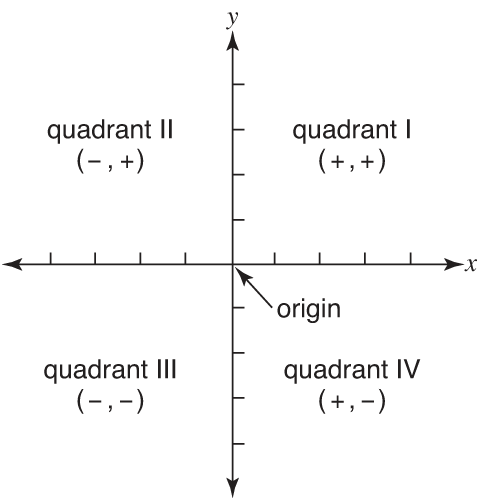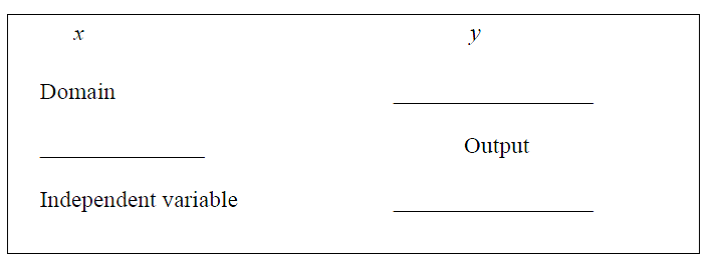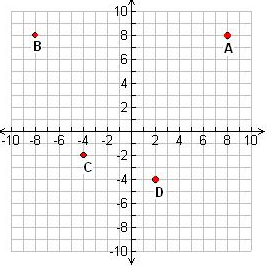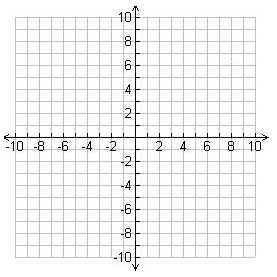Relationship between two variables

Relationship between two variables

This lesson will teach us the basic concepts of relationship between two quantities in the coordinate system. For instances, is y a dependent variable or independent variable? What is domain and range? What is an ordered pair? What is quadrant? And how do we plot points on a graph?

Lessons

Coordinate
System
: A presentation of a real number by points on the real number line using an order pair.

Order
pairs:
A point ( x , y ) on the coordinate plane. Eg. (-3,5), (0,5)…

Origin: The intersection of x-axis and y-axis $\to ( 0 , 0 )$

Relations: Sets of ordered pairs ( x , y )

Domain: All the x-values on the coordinate system

Range: All the y-values on the coordinate system

Independent Variable: The "x" values, which are also known as the inputs when solving for a solution.

Dependent Variable: The "y" values, which are also known as the outputs when solving for a solution.• 1.
Understanding the difference between X & Y
a)• 2.
Express the relation using mapping notation and ordered pairs.
a)
x = { -5 , -3 , -1 , 1 , 3 }
y = { 2 , 4 , 6 , 10 , 12 }

b)
x = { -4 , -2 , 0 , 2 , 4 }
y = { 3 , 3 , 3 , 3 , 3 }

c)
(1,3) , (2,2) , (3,1)

• 3.
Indicate the quadrant of the following points.
a)
(5, -3) (-3, 4) (-4, -3) (2, -6)

• 4.
Plot or find the points on the grid
a)
Find the coordinates A, B, C & D pointsb)
Plot the following points on the grid:
(4,5), (-2, 4), (-4,-3) & (5,-6)